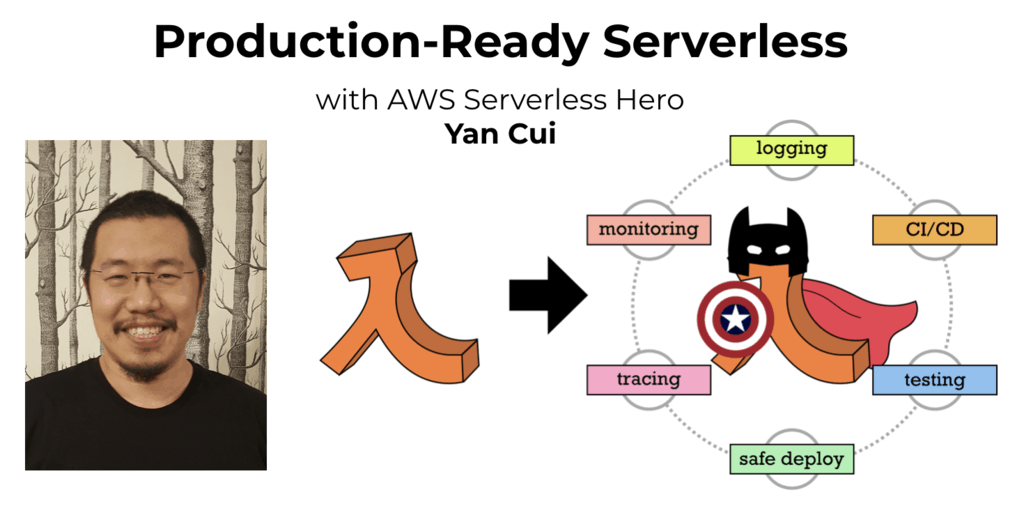# Project Euler – Problem 9 Solution

Check out my new course Learn you some Lambda best practice for great good! and learn the best practices for performance, cost, security, resilience, observability and scalability.

#### Problem

A Pythagorean triplet is a set of three natural numbers, a < b < c, for which,

a2 + b2 = c2

For example, 32 + 42 = 9 + 16 = 25 = 52.

There exists exactly one Pythagorean triplet for which a + b + c = 1000.

Find the product abc.

#### Solution

```let isPythagoreanTriplet(numbers : int list) =
match List.sort(numbers) with
| [a; b; c] -> a*a + b*b = c*c
| _ –> false

let getTriplets =
seq {
for a = 1 to 1000 do
for b = 1 to 1000 do
for c = 1 to 1000 do
if a + b + c = 1000 then yield [a; b; c]
}

let pythagoreanTriplet = getTriplets |> Seq.filter isPythagoreanTriplet |> Seq.head
let product = pythagoreanTriplet |> Seq.fold (fun acc x -> acc * x) 1
```

Here I’ve created a function called isPythagoreanTriplet, which takes a integer list and checks whether it’s a pythagorean triplet. If this is the first time you’ve seen the match …. with syntax, it’s used to match a pattern in F#. The patterns are evaluated from top to bottom, the first pattern decomposes a sorted version of the supplied int list into three values a, b and c, and evaluates whether a, b and c makes a pythagorean triplet:

```| [a; b; c] -> a*a + b*b = c*c
```

If the list does not have exactly three elements, then the next pattern is evaluate. The _ character is the wildcard character and in this case it simply returns false always. e.g.:

```isPythagoreanTriplet [3;4;5;0];;
val it : bool = false

isPythagoreanTriplet [];;
val it : bool = false

isPythagoreanTriplet [3;4;5];;
val it : bool = true
```

The getTriplets function on the hand, supplies a sequence of all triplets which matches the a + b + c = 1000 requirement. Note that I used the yield keyword to produce values that become part of the returned sequence.

The next line of code simply identifies the first (Seq.head) triplet from the above sequence which is a pythagorean triplet.

And finally, the product of the pythagorean triplet is calculated using the Seq.fold function (identical to the function used in the problem 8 solution, which describes the function in more detail).Hi, I’m Yan. I’m an AWS Serverless Hero and the author of Production-Ready Serverless.

I specialise in rapidly transitioning teams to serverless and building production-ready services on AWS.

Are you struggling with serverless or need guidance on best practices? Do you want someone to review your architecture and help you avoid costly mistakes down the line? Whatever the case, I’m here to help.Check out my new course, Learn you some Lambda best practice for great good! In this course, you will learn best practices for working with AWS Lambda in terms of performance, cost, security, scalability, resilience and observability. Enrol now and enjoy a special preorder price of £9.99 (~\$13).Are you working with Serverless and looking for expert training to level-up your skills? Or are you looking for a solid foundation to start from? Look no further, register for my Production-Ready Serverless workshop to learn how to build production-grade Serverless applications!